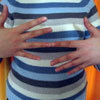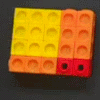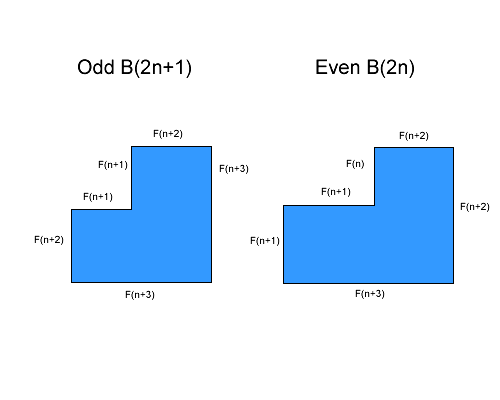#### You may also like### Building Gnomons

Build gnomons that are related to the Fibonacci sequence and try to explain why this is possible.### What Is the Question?

These pictures and answers leave the viewer with the problem "What is the Question". Can you give the question and how the answer follows?### Factorising with Multilink

Can you find out what is special about the dimensions of rectangles you can make with squares, sticks and units?

# Gnomon Dimensions

##### Age 14 to 16Challenge Level

1) Is there a pattern to the lengths and widths? Now look at the length and width of the rectangle cut out of each gnomon. Can you see any patterns here?

Jamie noticed some patterns:

The odd gnomons are a square with a little square taken out of it. All the sides are fibonacci numbers. The even gnomons are rectangles where the sides are consecutive fibonacci numbers, and the cut out corner is another rectangle made from consecutive fibonacci numbers. The bigger fibonacci number is the length, and the short one is the width.

Rosie develops this idea:

Using the patterns spotted by Yana in Building Gnomons, I found that the dimensions of a Gnomon are dependant on whether it is an odd or even Gnomon. In my diagram I used $B$ instead of $G$ to label a gnomon.So here $B(2n+1)$ is like $G_{2n+1}$ on the problem page, where it describes the number of the Gnomon. $F(n+1)$ means $F_{n+1}$ giving the $n+1 th$ fibonacci number, where the $2n+1$ refers to the number of the Gnomon.

So we can see there is a pattern, that the odd Gnomons are built around a square, if the Gnomon has number $2n+1$, then the gnomon is a sqaure of length and width $F_{n+3}$, with square of length $F_{n+1}$. For example if the Gnomon is size $9=2n+1=2\times 4 +1$. So the edge lengths are $F_{4+3}=F_7=13$, and the small square has edge length $F_{4+1}=F_5=5$.
So the area of the $9th$ Gnomon is $13\times 13 - 5\times 5 = 169 - 25 = 144$ which is the $12th$ fibonacci number.

For the even Gnomons, if the Gnomon is number $2n$, then the length and width are $F_{n+3}$ and $F_{n+2}$, and the small rectangle is $F_{n+1}$ by $F_n$. For example, if we take Gnomon number $8=2n=2\times 4$. So the area of the big rectangle is
$F_{n+3}\times F_{n+2} = F_7 \times F_6 = 13 \times 8 = 104$.
And the area of the little rectangle is
$F_{n+1}\times F_{n} = F_5 \times F_4 =5 \times 3 = 15$
So the area of the $8th$ Gnomon is $104-15=89$ which is the $11th$ fibonacci number.

2) The groups of gnomons with area 3, 8 and 21, and the gnomons with area 5, 13 and 34. Can you explain why I want to divide these into two separate groups? Can you give a convincing argument why all the gnomons fit into one of these two groups?

The first group are the areas of the odd Gnomons, those which are the square gnomons with square cut outs. The second group are the areas of the even Gnomons, which are based on rectangles.
Making these groups are useful because then they are divided into the groups in which they can be drawn.
All Gnomons will fit in one of these two groups, as all are odd or even.